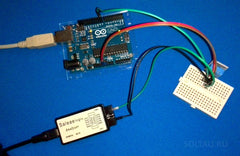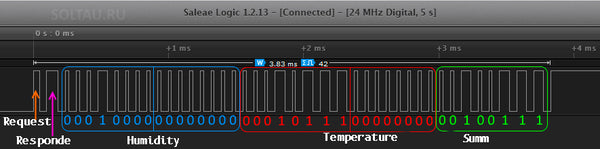# 🌤 How to connect the temperature and humidity sensor DHT11 to Arduino

DHT11 temperature and humidity sensor is a popular and cheap sensor that can be used in a fairly wide range of temperatures and relative humidity. Let's take a look at how to connect it to the Arduino and how to read data from it.

We will need:
• Arduino UNO or another compatible card;
• module with temperature and humidity sensor DHT11;
• connecting wires (for example, a good set);
• logic analyzer (optional);

Specifications of temperature and humidity sensor DHT11

So, the DHT11 sensor has the following characteristics:

• range of measured relative humidity - 20..90% with an error of up to 5%,
• The range of measured temperatures is 0..50 ° C with an error of up to 2 ° C;
• reaction time to changes in humidity - up to 15 seconds, temperatures - up to 30 seconds;
• The minimum polling period is 1 second.As you can see, the DHT11 sensor is not very precise, and the temperature range does not cover negative values, which is hardly suitable for outdoor measurements in the cold season in our climate. However, the low cost, small size, and ease of working with it partially overlap these disadvantages. The figure shows the appearance of the sensor and its dimensions in millimeters.

Wiring diagram for temperature and humidity sensor DHT11

Consider the connection diagram of the temperature sensor and humidity DHT11 to the microcontroller, in particular, to the Arduino.Let's see what is shown in the figure.

Designation in the figure Description Note
MCU Microcontroller or single board computer Arduino / Raspberry Pi etc
DHT11 Temperature and humidity sensor Pins 1Pin, 2Pin, and 4Pin are involved in the circuit, one of the sensor pins - 3rd pin 3Pin - is not connected to anything.
DATA Data bus If the length of the connecting cable from the sensor to the microcontroller does not exceed 20 meters, it is recommended to pull this bus to the power supply with a 5.1 kΩ resistor; if more than 20 meters, then another suitable value (smaller).
VDD Sensor supply Voltages from ~ 3.0 to ~ 5.5 volts DC are acceptable; if a power supply of ~ 3.3 V is used, then it is desirable to use a power wire no longer than 20 cm.

Let's collect the considered scheme. I will also traditionally include a logic analyzer in the circuit so that you can study the timing diagram of information exchange with the sensor.The DHT11 sensor is often sold as a complete assembly with the necessary strapping — a pull-up resistor and a filter capacitor (as in the previous photo). For experiments with Arduino, I recommend buying just that.

Reading data from a DHT11 sensor using Arduino

Let's go this way: download the library for the DHT11 sensor, install it in the standard way (by unpacking into the \ libraries \ directory of the development environment for Arduino).

Let's write such a simple sketch. It will output to the serial port of the computer every 2 seconds messages about relative humidity and temperature, read from the DHT11 sensor.

```#include <dht11.h>
dht11 sensor;
#define DHT11PIN 8

void setup() {
Serial.begin(9600);
}

void loop() {

Serial.print("h=");
Serial.print(sensor.humidity);
Serial.print("%\t");

Serial.print("t=");
Serial.print(sensor.temperature);
Serial.println("C");

delay(2000);
}```

Load this sketch into Arduino. Connect to the Arduino using the monitor of the COM port and see the following:It is seen that data on both humidity and temperature are read and output to the terminal.

The timing diagram of information exchange of a temperature and humidity sensor DHT11 with a microcontroller

With the help of the timing diagram obtained from the logic analyzer, let us examine how information exchange is carried out.

The DHT11 temperature and humidity sensor use a single-wire serial packet interface to communicate with the microcontroller. One information packet with a duration of about 4 ms contains 1 request bit from the microcontroller, 1 sensor response bit and 40 data bits from the sensor (16 bits of humidity information, 16 bits of temperature information, and 8 check bits). Let's take a closer look at the Arduino timeline information flow chart with a DHT11 sensor.The figure shows that there are two types of pulses: short and long. Short ones in this exchange protocol denote zeros, long pulses - ones.

So, the first two pulses are the Arduino's request to the DHT11 and, accordingly, the response of the sensor. Next, come 16 bits of moisture. And they are divided into bytes, senior and junior, senior left. That is, in our figure, the humidity data is as follows:
0001000000000000 = 00000000 00010000 = 0x10 = 16% relative humidity.

Temperature data is similar:
0001011100000000 = 00000000 00010111 = 0x17 = 23 degrees Celsius.

The checksum is just an arithmetic summation of 4 received data bytes:
00000000 +
00010000 +
00000000 +
00010111 =
00100111 in binary or 0 + 16 + 0 + 23 = 39 in decimal.

Using the DHT11 sensor without a library

Now we know enough to write our own program for working with the temperature and humidity sensor DHT11 without using third-party libraries. We will write a sketch that will poll the sensor once a second and output the received packet and data on temperature, humidity, and also the check byte to the serial port of the computer. On the 13th leg of the Arduino, we will derive a control signal and, having connected in it with a logic analyzer, we will check that we correctly read the information from the sensor.

#define DHT11pin 8
#define LEDpin 13
void setup() {
Serial.begin(9600);
pinMode(DHT11pin, INPUT);
pinMode(LEDpin, OUTPUT);
}
void loop() {
}
else {
delay(1000);
processDht11Data();
resetVals();
}
}
pinMode(DHT11pin, OUTPUT);
digitalWrite(DHT11pin, LOW);
delay(15);
pinMode(DHT11pin, INPUT);
}
digitalWrite(LEDpin, sensorValue);
}
void processDht11Data() {
byte dht11Data = {0,0,0,0,0,0,0,0,0,0,0,0,0,0,0,0,0,0,0,0,0,0,0,0,0,0,0,0,0,0,0,0,0,0,0,0,0,0,0,0,0};
int zeroLen = 1;
int oneLen = 3 * zeroLen;
int wrongData = 6 * zeroLen;
int currentBitLen = 0;
int bitPosition = 0;
for (int i=1; i= zeroLen) && (currentBitLen <= oneLen)) {
dht11Data[bitPosition] = 0;
bitPosition++;
}
else if ((currentBitLen > oneLen) && (currentBitLen <= wrongData)) {
dht11Data[bitPosition] = 1;
bitPosition++;
}
currentBitLen = 0;
}
else {
currentBitLen += 1;
}
}
}
for (int i=0; i<41; i++) {
Serial.print(dht11Data[i]);
Serial.print(" ");
}
Serial.println();
getHumidTemperatureParity(dht11Data);
}
void getHumidTemperatureParity(byte data[]) {
word humidity = 0;
byte hLow = 0;
byte hHi = 0;
word temperature = 0;
byte tLow = 0;
byte tHi = 0;
byte parity = 0;
for (int i=1; i<9; i++){
hLow = hLow | (data[i] << (8 - i));
}
for (int i=9; i<17; i++){
hHi = hHi | (data[i] << (16 - i));
}
for (int i=17; i<25; i++){
tLow = tLow | (data[i] << (24 - i));
}
for (int i=25; i<33; i++){
tHi = tHi | (data[i] << (32 - i));
}
for (int i=33; i<41; i++){
parity = parity | (data[i] << (40 - i));
}
humidity = word(hHi, hLow);
temperature = word(tHi, tLow);
Serial.print("h=");
Serial.print(humidity);
Serial.print("%\t");
Serial.print("t=");
Serial.print(temperature);
Serial.print("C\t");
Serial.print("parity=");
Serial.println(parity);
}
void resetVals() {
}
A small table will give additional explanations to the proposed solution.

Function Purpose Comment
initLink() Sends a pulse to initiate an exchange with a DHT11. In INPUT mode, the Arduino digital pins are in the "1" state. We transfer them to the OUTPUT mode and for 15 ms we lower them to "0", which serves as a signal of the beginning of the exchange for the DHT11 sensor.
readSerialDHT11() Reads the DHT11 sensor data and writes to the array. We constantly poll the state of the DATA line, write the received data to the array (up to the top of the array), display the received data to check on the 13th pin of the Arduino (I needed this for debugging, in the “clean” code this piece can be removed).
processDht11Data() Processes an array of data taken in one cycle (one packet). On the leading edges of received pulses, we fix the fact of the incoming new bit and consider its duration in arbitrary units. For the duration of the estimated - it is a bit unit or bit-zero (in some specified limits). We make a package of 41 bits.
getHumidTemperatureParity() Receives data on humidity, temperature from the package. Select from 41 bits of the packet data on humidity, temperature, and checksum.
resetVals() Resets variables to their original state. With the expansion of the program there may appear more variables that require a reset.

This is what the timeline will look like. Here, A is the signal on the DATA bus of the DHT11 sensor, B is the readable signal.It can be seen that we read the data with a slight delay. In addition, we begin to read the data from the response signal and skip the request signal. We do not take into account this first impulse in the program since it does not relate directly to the data. In the output of a COM port monitor, we can observe a similar pattern: a long string of ones and zeros are our packets, and it can be seen that they all start with a single bit since we read packets starting at the response bit.What we did not take into account in this program is that the minimum duration of a bit-zero pulse can vary within certain limits, and we have rigidly specified its value in the code. For good, you still need to write the function getMinimalBitLen (), which would find the minimum duration (in arbitrary units) of one bit-zero in the packet being processed.